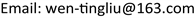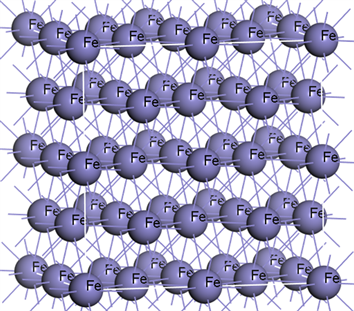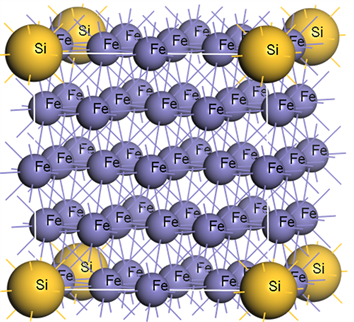﻿ Fe-X (X = Si, Cr, Mn, Ni)二元合金结构和弹性性质的第一性原理计算 First-Principles Study on Structure and Elastic Properties of Fe-X (X = Si, Cr, Mn, Ni) Binary Alloy

Material Sciences
Vol.07 No.06(2017), Article ID:22123,6 pages
10.12677/MS.2017.76080

First-Principles Study on Structure and Elastic Properties of Fe-X (X = Si, Cr, Mn, Ni) Binary Alloy

Wenting Liu, Xuan Yang, Man Li, Chen Chen, Kai Zhao

School of Materials Science and Engineering, Xi’an Shiyou University, Xi’an ShaanxiReceived: Aug. 21st, 2017; accepted: Sep. 14th, 2017; published: Sep. 21st, 2017ABSTRACT

The effects of alloying element such as Si, Cr, Mn and Ni on the structure and elastic properties of face centered cubic Fe were investigated by the method of the first-principles based on density functional theory. The results showed that the alloying elements (Si, Cr, Mn, Ni) were all increased the lattice constant of face centered cubic Fe, and thus increased the cell volume. After adding the alloying elements, the all binary alloy systems meet the mechanical stability conditions. The elastic constants of C11, C12, and C44 were increased almost all, the elastic moduli were increased also and the Poisson’s ratio was changed little as a whole.

Keywords:

Fe-X (X = Si, Cr, Mn, Ni) Binary Alloy, Elastic Properties, Structure, First-Principles

Fe-X (X = Si, Cr, Mn, Ni)二元合金结构和弹性性质的第一性原理计算1. 引言

2. 计算方法和模型

Voigt模型中，体模量Bv和剪切模量Gv与弹性常数的关系为：

${B}_{V}=\frac{1}{3}\left({C}_{11}+2{C}_{12}\right)$ (1)

${G}_{V}=\frac{1}{15}\left({C}_{11}-{C}_{12}+3{C}_{44}\right)$ (2)

${B}_{R}=\frac{1}{3{S}_{11}+6{S}_{12}}$ (3)

${G}_{R}=15{\left[4\left({S}_{11}-{S}_{12}\right)+3{S}_{44}\right]}^{-1}$ (4)

${B}_{V}={B}_{R}=\frac{1}{3}\left({C}_{11}+2{C}_{12}\right)$ (5)

${G}_{R}=\frac{15\left({C}_{11}-{C}_{12}\right){C}_{44}}{4{C}_{44}+3\left({C}_{11}-{C}_{12}\right)}$ (6)

Hill模型的体模量B和剪切模量G可以通过将Voigt和Reuss模型的计算结果进行平均值简化得到，即：

$B=\frac{1}{2}\left({B}_{V}+{B}_{R}\right)$ (7)

$G=\frac{1}{2}\left({G}_{V}+{G}_{R}\right)$ (8)

$E=\frac{9BG}{3B+G}$ (9)

$v=\frac{3B-2G}{2\left(3B+G\right)}$ (10)

3. 结果与讨论

3.1. 晶体结构

3.2. 弹性性质(a)(b)

Figure 1. The unit cell structure of Fe32(a) and Fe31Si(b)Table 1. The lattice parameter of Fe and Fe-X(X = Si, Cr, Mn, Ni) binary alloyTable 2. The calculated elastic constants of Fe and Fe-X(X = Si, Cr, M, Ni) binary alloyTable 3. Calculated bulk, shear and Young’s modulus and Poisson coefficient for Fe and Fe-X(X = Si, Cr, Mn, Ni) binary alloy

4. 结论

First-Principles Study on Structure and Elastic Properties of Fe-X (X = Si, Cr, Mn, Ni) Binary Alloy[J]. 材料科学, 2017, 07(06): 609-614. http://dx.doi.org/10.12677/MS.2017.76080

1. 1. 吴承建, 陈国良, 强文江. 金属材料学[M]. 北京: 冶金工业出版社, 2009.

2. 2. 户秀萍, 张彩丽, 王小, 张齐娜, 董楠, 韩培德. Fe-X(Mn,Si)合金稳定性和弹性性能的第一性原理研究[J]. 太原理工大学学报, 2013, 44(6): 683-688.

3. 3. 沈莲. 机械工程材料[M]. 北京: 机械工业出版社, 2007.

4. 4. 赵莉萍. 金属材料学[M]. 北京: 北京大学出版社, 2012.

5. 5. 李具仓, 陈银莉, 汪淑英, 赵爱民, 王丽娜. 一种新型的含镍耐蚀高硅铁基合金[J]. 北京科技大学学报, 2007, 29(2): 125-129.

6. 6. 王东, 曹建春, 周晓龙, 邓蕾. 钢铁材料的第一性原理研究进展[J]. 材料导报, 2013, 27(2): 124-126.

7. 7. Hill, R. (1952) The Elastic Behavior of a Crystalline Aggregate. Proceedings of the Physical Society, 65, 349-354. https://doi.org/10.1088/0370-1298/65/5/307

8. 8. 汪向荣. AerMet100钢热变形行为与热处理组织性能及其第一性原理表征[D]: [博士学位论文]. 哈尔滨: 哈尔滨工业大学, 2009.

9. 9. Li, L.Y., Yu, W. and Jin, C.Q. (2005) First-Principles Calculations of a High-Pressure Synthesized Compound PtC. Physics Condensed Matter, 17, 5965-5970. https://doi.org/10.1088/0953-8984/17/38/002

10. 10. 曾宪波, 彭平. α2-Ti-25Al-xNb合金力学性质的第一原理计算[J]. 金属学报, 2009, 45(9): 1049-1056.

11. 11. Bannikov, V.V., SHEIN, I.R. and Ivanovskii, A.L. (2012) Structural, Electronic, Magnetic and Elastic Properties of Teragonal Layered Diselenide KCo2Se2 from First Principles Calculations. Physica B: Condensed Matter, 407, 271- 275. https://doi.org/10.1016/j.physb.2011.10.046

12. 12. 童立甲. Fe-Mn合金的弹性性能和电子结构的研究[D]: [硕士学位论文]. 唐山: 河北联合大学, 2014.